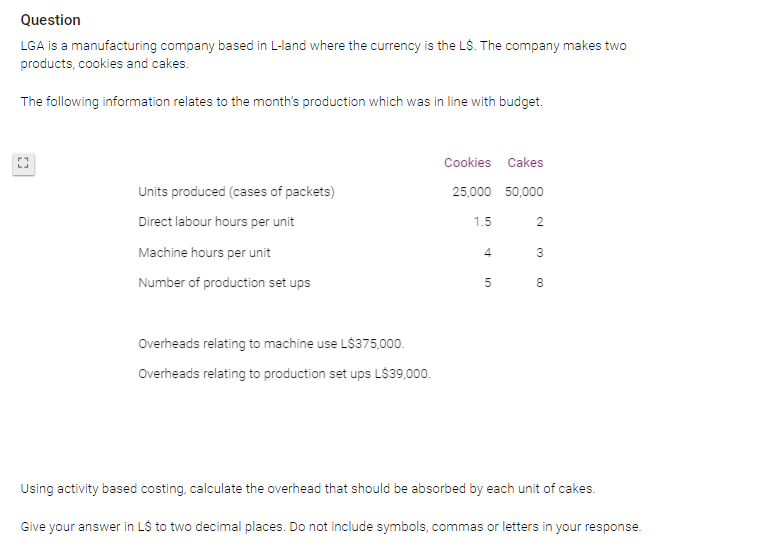# ABC Costing

Brushing up on ABC costing and this question has thrown me off. Maybe it was the way it was written or something isn't clicking.

Can anyone help?• The "activity based costs " we are interested in are the overhead cost related to machine use and the overhead cost related to set ups.

The key calculation for ABC is usually the cost driver rate (https://docs.google.com/document/d/1icNhvz1m0pMF3CF_oVZfQAo36QBn2qmxmiWndjFECYg/edit?usp=sharing )

In this case these are:
Machine use: L\$375,000/machine hours (see below) = L\$1.027 per hour
and
Set ups: L\$39,000/number of sets ups (see below) = L\$0.074

Machine hours = (9 x 25,000) + (3/50,000) = 365,000 hrs
Set ups = (5 x 25,000) + (8 x 50,000) = 525,000

Cakes: 50,000 x 3hrs = 150,000 machine hours costing 150,000 x 1.027 = L\$154,050
50,000 x 8setups = 400,000 setups costing 400,000 x 0.074 = L\$29,600
Total = 154,050 + 29,600 = L\$183,650

Overhead per cake = 183,650/50,000 = L\$3.67
• That’s hard.
ABC costing is budgeted overheads/direct labour or direct machine hour (BOAR) times the actual and compare to the actual. But this question….